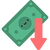top of page### Aggregate Expenditure Model

#### Multiplier Process

###### Content ContributorsChristian Bien

# Learning Objectives###### ​###### What is the Multiplier?In economics, the multiplier follows the change in real GDP from a given change in one component of aggregate expenditure. Think about the Elizabeth Quay project, the initial cost for the Government is \$440 million. So initially, the Elizabeth Quay project will provide an injection of \$440 million in the WA economy. However, the multiplier considers the effect of one person's spending becoming another person's income. So the people employed in the Elizabeth Quay project (engineers, builders, landscapers, planners) will spend their incomes on other things such as food, leisure, fashion and so on. Their spending will provide other people's income. A proportion of the income is saved and the remainder spent providing another set of people's income and so on. Each time the effect of increases in real GDP decreases until it becomes 0. Hence, an initial change in expenditure (in this case Government expenditure) will always cause a greater change in real GDP due to a flow-on effect.###### ​###### ​###### What Causes the Size of the Multiplier?We have to remember that when we have an increase in expenditure that not all of it is spent. In a simple of the economy of only a finance industry, we have to consider the effect of the proportion of income that is saved and not spent.###### Calculating the Simple MultiplierThe multiplier requires a MPC or MPS.

Multiplier (k) = 1/MPS OR (Change in RGDP)/(Change in Consumption)

Remember that MPS = 1 - MPC###### Can You Calculate the Simple Multiplier from the Below Model?Method 1

Change in RGDP/Change in Consumption Multiplier (K) = 800/400

= 2 Multiplier = 2

Method 2

Calculating MPC MPC = Change in Consumption/ Change in RGDP =400/800 =0.5

MPS = 1 - 0.5 = 0.5

Multiplier (K) = 1/0.5 = 2###### The Complex MultiplierWe also have to remember that savings is not the only leakage. In the full circular flow model, we remember that the leakages are savings, taxation and imports. For example, consider the effect of the Elizabeth Quay project. The employees hired for the project will spend their incomes, but not all of it as a portion is saved, a portion is paid in income taxes and a bit is spent on imports. The remaining amount is spent as consumption providing other people's income, who then save, pay taxes and import and whatever leftover is spent as consumption for other people's income and so on... Each time the flow-on effect of income decreases until it becomes eventually 0. Therefore, when we have the multiplier effect, we also got to consider the decrease in the flow of income from savings, taxation and imports.

Hence, the full multiplier formula is:

Multiplier (k) = 1/(MPS + MPT + MPM)

MPS = Marginal Propensity to Save MPT = Marginal Propensity to Tax (increase in the amount of tax paid for an increase in income) MPM = Marginal Propensity to Import (increase in the number of imports for an increase in income)###### ​bottom of page